【我們為什麼挑選這篇文章】從比特幣被發明至今，已有無數自稱專家的人出面，跟大家解釋何謂比特幣，未來發展如何，但這些論述往往缺乏數據的背書，難以驗證。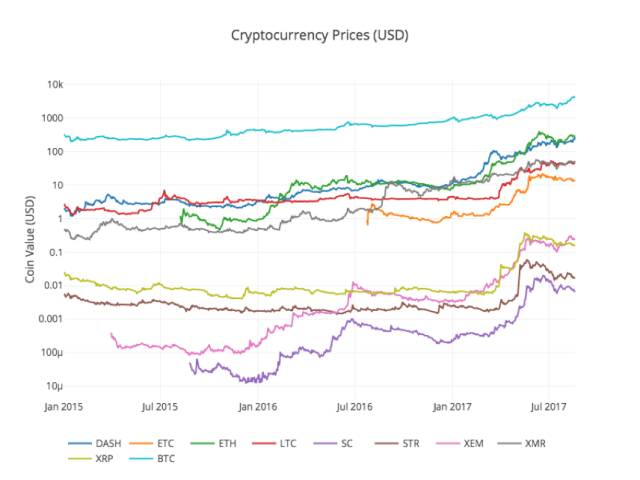## 步驟1 – 配置你的數據實驗室

Ananconda會為每一個項目的依賴包創建一個特殊的環境目錄，使得所有包都能妥善地被管理和區分。

##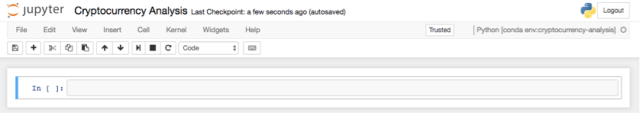import os import numpy as np import pandas as pd import pickle import quandl from datetime import datetime

import plotly.offline as py import plotly.graph_objs as go import plotly.figure_factory as ff py.init_notebook_mode(connected=True)

## 步驟2 – 獲得比特幣的價格數據

def get_quandl_data(quandl_id): ”’Download and cache Quandl dataseries”’ cache_path = ‘{}.pkl’.format(quandl_id).replace(‘/’,’-‘) try: f = open(cache_path, ‘rb’) df = pickle.load(f) print(‘Loaded {} from cache’.format(quandl_id)) except (OSError, IOError) as e: print(‘Downloading {} from Quandl’.format(quandl_id)) df = quandl.get(quandl_id, returns=”pandas”) df.to_pickle(cache_path) print(‘Cached {} at {}’.format(quandl_id, cache_path)) return df

# Pull Kraken BTC price exchange data btc_usd_price_kraken = get_quandl_data(‘BCHARTS/KRAKENUSD’)

# Chart the BTC pricing data btc_trace = go.Scatter(x=btc_usd_price_kraken.index, y=btc_usd_price_kraken[‘Weighted Price’]) py.iplot([btc_trace])

# Pull pricing data for 3 more BTC exchanges exchanges=[‘COINBASE’,’BITSTAMP’,’ITBIT’]exchange_data={}exchange_data[‘KRAKEN’]=btc_usd_price_kraken for exchange in exchanges:exchange_code=’BCHARTS/{}USD’.format(exchange)btc_exchange_df=get_quandl_data(exchange_code)exchange_data[exchange]=btc_exchange_df

##### 步驟2.4 將所有價格數據整合到單一數據框之中

def merge_dfs_on_column(dataframes, labels, col):”’Merge a single column of each dataframe into a new combined dataframe”’series_dict={} for index in range(len(dataframes)):series_dict[labels[index]]=dataframes[index][col]return pd.DataFrame(series_dict)

# Merge the BTC price dataseries’ into a single dataframebtc_usd_datasets=merge_dfs_on_column(list(exchange_data.values()), list(exchange_data.keys()),’Weighted Price’)

btc_usd_datasets.tail()

def df_scatter(df, title,seperate_y_axis=False,y_axis_label=”, scale=’linear’,initial_hide=False):”’Generate a scatter plot of the entire dataframe”’label_arr= list(df)series_arr= list(map(lambda col:df[col],label_arr)) layout =go.Layout(title=title, legend=dict(orientation=”h”), xaxis=dict(type=’date’), yaxis=dict(title=y_axis_label, showticklabels=notseperate_y_axis, type=scale))y_axis_config=dict(overlaying=’y’, showticklabels=False, type=scale ) visibility =’visible’ifinitial_hide: visibility =’legendonly’# Form Trace For Each Seriestrace_arr=[]for index, series in enumerate(series_arr): trace =go.Scatter(x=series.index, y=series, name=label_arr[index], visible=visibility)# Add seperate axis for the seriesif seperate_y_axis: trace[‘yaxis’]=’y{}’.format(index +1) layout[‘yaxis{}’.format(index +1)]=y_axis_configtrace_arr.append(trace) fig =go.Figure(data=trace_arr, layout=layout)py.iplot(fig)

# Plot all of the BTC exchange pricesdf_scatter(btc_usd_datasets,’Bitcoin Price (USD) By Exchange’)

# Remove “0” valuesbtc_usd_datasets.replace(0,np.nan,inplace=True)

# Plot the revised dataframedf_scatter(btc_usd_datasets,’Bitcoin Price (USD) By Exchange’)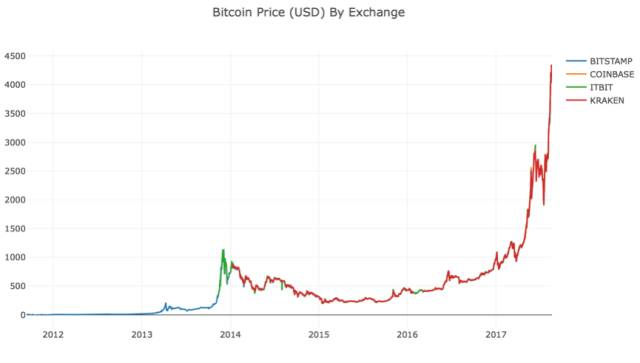# Calculate the average BTC price as a new columnbtc_usd_datasets[‘avg_btc_price_usd’]=btc_usd_datasets.mean(axis=1)

# Plot the average BTC pricebtc_trace=go.Scatter(x=btc_usd_datasets.index, y=btc_usd_datasets[‘avg_btc_price_usd’])py.iplot([btc_trace])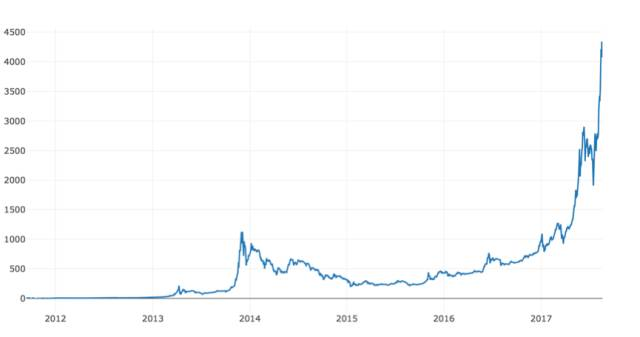## 步驟3 獲取山寨幣（Altcoins）價格

##### 步驟3.1 定義Poloniex交易所的API輔助函數

base_polo_url=’https://poloniex.com/public?command=returnChartData&currencyPair={}&start={}&end={}&period={}’start_date=datetime.strptime(‘2015-01-01′,’%Y-%m-%d’)# get data from the start of 2015end_date=datetime.now()# up until todaypediod=86400# pull daily data (86,400 seconds per day)def get_crypto_data(poloniex_pair):”’Retrieve cryptocurrency data from poloniex”’json_url=base_polo_url.format(poloniex_pair,start_date.timestamp(),end_date.timestamp(),pediod)data_df=get_json_data(json_url,poloniex_pair)data_df=data_df.set_index(‘date’)return data_df

##### 步驟3.2 從Poloniex下載交易數據

altcoins=[‘ETH’,’LTC’,’XRP’,’ETC’,’STR’,’DASH’,’SC’,’XMR’,’XEM’]altcoin_data={} for altcoin in altcoins:coinpair=’BTC_{}’.format(altcoin)crypto_price_df=get_crypto_data(coinpair)altcoin_data[altcoin]=crypto_price_df

altcoin_data[‘ETH’].tail()##### 步驟3.3 – 統一貨幣單位：美元

# Calculate USD Price as a new column in each altcoin dataframe for altcoin in altcoin_data.keys(): altcoin_data[altcoin][‘price_usd’] = altcoin_data[altcoin][‘weightedAverage’] * btc_usd_datasets[‘avg_btc_price_usd’]

# Merge USD price of each altcoin into single dataframe combined_df = merge_dfs_on_column(list(altcoin_data.values()), list(altcoin_data.keys()), ‘price_usd’)

# Add BTC price to the dataframe combined_df[‘BTC’] = btc_usd_datasets[‘avg_btc_price_usd’]

# Chart all of the altocoin prices df_scatter(combined_df, ‘Cryptocurrency Prices (USD)’, seperate_y_axis=False, y_axis_label=’Coin Value (USD)’, scale=’log’)##### 步驟3.4- 執行相關性分析

8/22/2017修訂說明-這部分的修改是為了在計算相關係數時使用每日回報率而不是價格的絕對值。

# Calculate the pearson correlation coefficients for cryptocurrencies in 2016 combined_df_2016 = combined_df[combined_df.index.year == 2016] combined_df_2016.pct_change().corr(method=’pearson’)def correlation_heatmap(df, title, absolute_bounds=True): ”’Plot a correlation heatmap for the entire dataframe”’ heatmap = go.Heatmap( z=df.corr(method=’pearson’).as_matrix(), x=df.columns, y=df.columns, colorbar=dict(title=’Pearson Coefficient’), ) layout = go.Layout(title=title) if absolute_bounds: heatmap[‘zmax’] = 1.0 heatmap[‘zmin’] = -1.0 fig = go.Figure(data=[heatmap], layout=layout) py.iplot(fig) correlation_heatmap(combined_df_2016.pct_change(), “Cryptocurrency Correlations in 2016”)combined_df_2017 = combined_df[combined_df.index.year == 2017] combined_df_2017.pct_change().corr(method=’pearson’)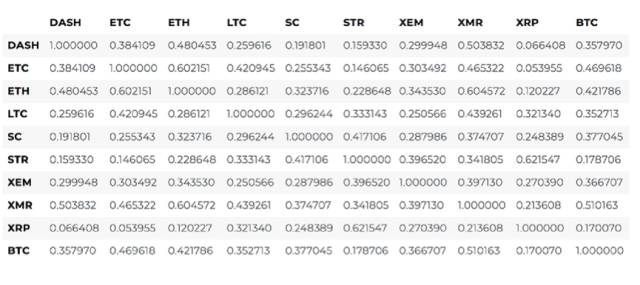correlation_heatmap(combined_df_2017.pct_change(), “Cryptocurrency Correlations in 2017”)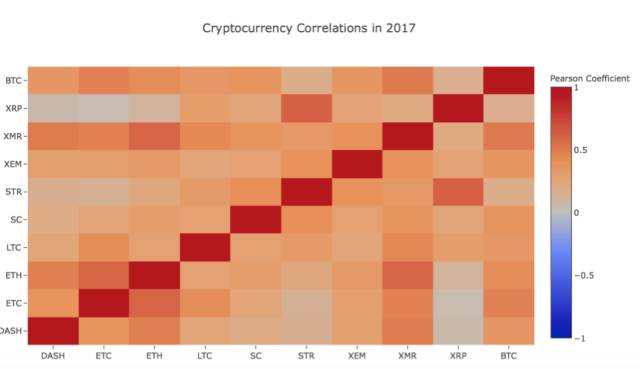## 現在，到你了！

• 為整個分析添加更多加密貨幣的數據
• 調整相關性分析的時間範圍和顆粒度，以得到優化的或粗粒度的趨勢視圖。
• 從交易量或區塊鏈數據挖掘集中尋找趨勢。相較於原始的價格數據，如果你想預測未來價格波動，你可以更需要買/賣量的比率數據。
• 在股票、商品、法定貨幣上加入價格數據來決定他們當中哪一項與電子貨幣具有相關性（但是，別忘了那句老話「相關不蘊含因果」）